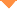首页 > 论文 > 中国激光 > 47卷 > 10期(pp：1006004--1)

Intrusion Event Identification for Fiber Perimeter Security System Based on ARMA Modeling and Sigmoid Fitting

• 摘要• 论文信息• 参考文献• 被引情况• PDF全文Abstract

In a practical optical fiber perimeter security system, not only the discrimination of multiple events but also the comprehensive probability evaluation of these events is required. Therefore, this paper proposes a recognition scheme combining autoregressive moving average (ARMA) modeling with Sigmoid probability fitting. In event discrimination, both the ARMA coefficients and the zero-crossing rate of an optical fiber vibration signal are incorporated into a feature vector, which is then fed into a support vector machine (SVM) to recognize six types of common intrusion events: climbing, knocking, waggling, cutting, kicking, and crashing. In comprehensive probability evaluation, the SVM training pattern outputs are used to fit the parameters of a Sigmoid function. Then, the SVM outputs of the test patterns are substituted into this fitted Sigmoid model to yield the expected result. Field experiments reveal that the average recognition rate of six intrusion events by the proposed scheme reaches 87.14%. Moreover, the occurrence probabilities of all intrusion events can be provided as references, thereby presenting vast potential for future applications.【1】Liu X, Jin B Q, Bai Q, et al. Distributed fiber-optic sensors for vibration detection [J]. Sensors. 2016, 16(8): 1164.Liu X, Jin B Q, Bai Q, et al. Distributed fiber-optic sensors for vibration detection [J]. Sensors. 2016, 16(8): 1164.

【2】Postolache O A, Faria J A B, et al. An overview and a contribution to the optical measurement of linear displacement [J]. IEEE Sensors Journal. 2001, 1(4): 322-331.

【3】Gong H P, Song H F, Zhang S L, et al. Curvature sensor based on hollow-core photonic crystal fiber Sagnac interferometer [J]. IEEE Sensors Journal. 2014, 14(3): 777-780.

【4】Sun Q Z, Liu D M, Liu H R, et al. Distributed disturbance sensor based on a novel Mach-Zehnder interferometer with a fiber-loop [J]. Proceedings of SPIE. 2005, 6344: 63440K.

【5】Liu D, Shum P. Distributed fiber-optic sensor with a ring Mach-Zehnder interferometer [J]. Proceedings of SPIE. 2007, 6781: 67814D.

【6】Yu S Y, Sun S L. The design of multi-wireless infrared detectors and intelligent burglary-resisting system [J]. Laser & Infrared. 2008, 38(4): 345-347.

【7】Fan Z M. Design principle and application status of tension electronic fence perimeter protection alarm system China Security & Protection[J]. 0, 2008(3): 42-45.

【8】Xie S R, Zhang M, Lai S R, et al. Positioning method for dual Mach-Zehnder interferometric submarine cable security system [J]. Proceedings of SPIE. 2010, 7677: 76770A.

【9】Jiang L H, Yang R Y. Identification technique for the intrusion of airport enclosure based on double Mach-Zehnder interferometer [J]. Journal of Computers. 2012, 7(6): 1453-1459.

【10】Juarez J C, Maier E W, Choi K N, et al. Distributed fiber-optic intrusion sensor system [J]. Journal of Lightwave Technology. 2005, 23(6): 2081-2087.

【11】Xie S R, Zou Q L, Wang L W, et al. Positioning error prediction theory for dual Mach-Zehnder interferometric vibration sensor [J]. Journal of Lightwave Technology. 2011, 29(3): 362-368.

【12】Huang X D, Yu J, Liu K, et al. Configurable filter-based endpoint detection in DMZI vibration system [J]. IEEE Photonics Technology Letters. 2014, 26(19): 1956-1959.

【13】Liu L, Sun W, Zhou Y, et al. Security event classification method for fiber-optic perimeter security system based on optimized incremental support vector machine . [C]∥Li S, Liu C, Wang Y. Pattern Recognition, CCPR 2014. Communications in Computer and Information Science. Berlin, Heidelberg: Springer. 2014, 484: 595-603.

【14】Qu H Q, Zheng T, Pang L P, et al. A new detection and recognition method for optical fiber pre-warning system [J]. Optik. 2017, 137: 209-219.

【15】Liu K, Tian M, Liu T G, et al. A high-efficiency multiple events discrimination method in optical fiber perimeter security system [J]. Journal of Lightwave Technology. 2015, 33(23): 4885-4890.

【17】Li K Y, Zhao X Q, Sun X H, et al. A regular composite feature extraction method for vibration signal pattern recognition in optical fiber link system [J]. Acta Physica Sinica. 2015, 64(5): 054304.

【18】Huan H X. Hien D T T, Tue H H. Efficient algorithm for training interpolation RBF networks with equally spaced nodes [J]. IEEE Transactions on Neural Networks. 2011, 22(6): 982-988.

【19】Lyons W B, Ewald H, Lewis E. An optical fibre distributed sensor based on pattern recognition [J]. Journal of Materials Processing Technology. 2002, 127(1): 23-30.

【21】Huang S J, Shih K R. Short-term load forecasting via ARMA model identification including non-Gaussian process considerations [J]. IEEE Transactions on Power Systems. 2003, 18(2): 673-679.

【22】Erdem E, Shi J. ARMA based approaches for forecasting the tuple of wind speed and direction [J]. Applied Energy. 2011, 88(4): 1405-1414.

【23】Chen Q N, Liu T G, Liu K, et al. An elimination method of polarization-induced phase shift and fading in dual Mach-Zehnder interferometry disturbance sensing system [J]. Journal of Lightwave Technology. 2013, 31(19): 3135-3141.

【24】Huang X D, Wang Y D, Liu K, et al. Event discrimination of fiber disturbance based on filter bank in DMZI sensing system [J]. IEEE Photonics Journal. 2016, 8(3): 1-14.

【26】Smola A J, Bartlett P, Sch?lkopf B, et al. Probabilities for SV machines[M]. Cambridge: , 2000, 61-74.

Huang Xiangdong,Wang Biyao,Liu Kun,Liu Tiegen. Intrusion Event Identification for Fiber Perimeter Security System Based on ARMA Modeling and Sigmoid Fitting[J]. Chinese Journal of Lasers, 2020, 47(10): 1006004